• +91 9971497814
• info@interviewmaterial.com

# RD Chapter 30- Derivatives Ex-30.5 Interview Questions Answers

### Related Subjects

Question 1 : Differentiate the following functions with respect to x: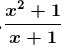Let us consider

y =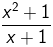We need to find dy/dx

We know that y is a fraction of two functions say u and v where,

u = x2 +1 and v = x + 1

y= u/v

Now let us apply quotient rule of differentiation.

By using quotient rule, we get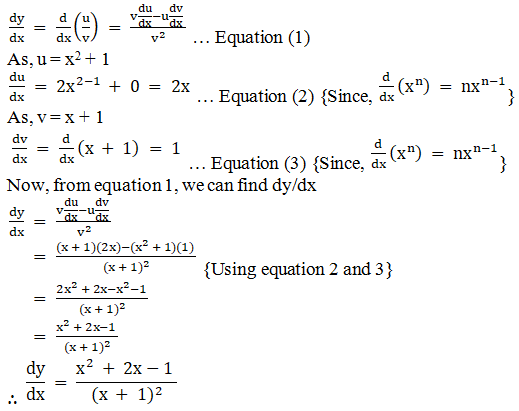Question 2 :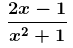Let us consider

y =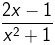We need to find dy/dx

We know that y is a fraction of two functions say u and v where,

u = 2x – 1 and v = x2 +1

y= u/v

Now let us apply quotient rule of differentiation.

By using quotient rule, we get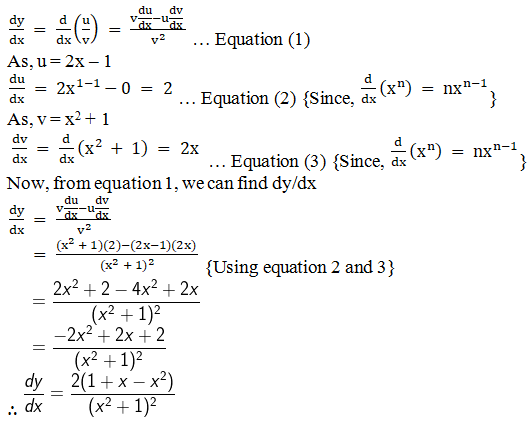Question 3 :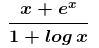Let us consider

y =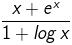We need to find dy/dx

We know that y is a fraction of two functions say u and v where,

u = x + ex and v= 1 + log x

y= u/v

Now let us apply quotient rule of differentiation.

By using quotient rule, we get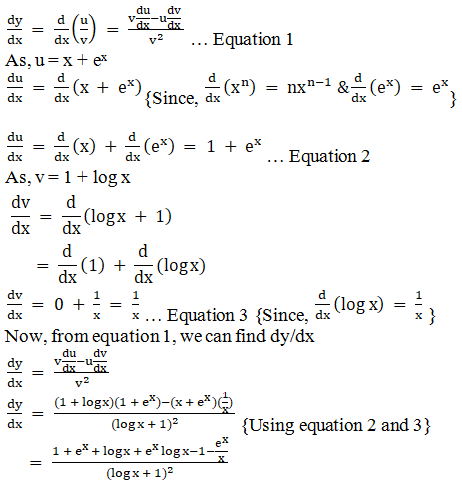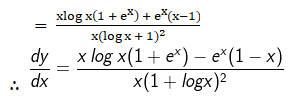Question 4 :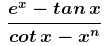Let us consider

y =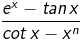We need to find dy/dx

We know that y is a fraction of two functions say u and v where,

u = ex – tanx and v = cot x – xn

y= u/v

Now let us apply quotient rule of differentiation.

By using quotient rule, we get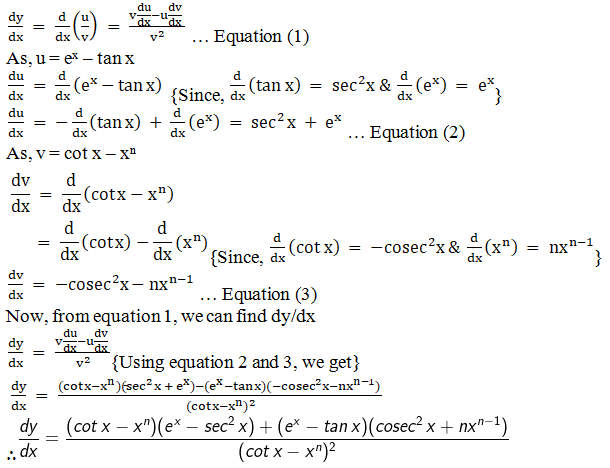Question 5 :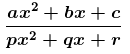Let us consider

y =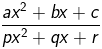We need to find dy/dx

We know that y is a fraction of two functions say u and v where,

u = ax2 + bx +c and v = px2 + qx + r

y= u/v

Now let us apply quotient rule of differentiation.

By using quotient rule, we get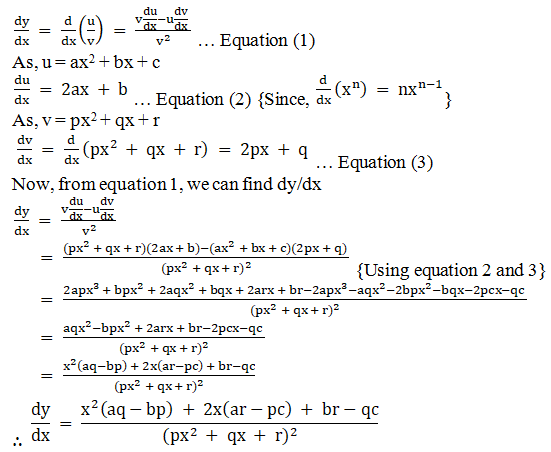Todays Deals### RD Chapter 30- Derivatives Ex-30.5 Contributorskrishan

Name:
Email:

# Latest News# 9000 interview questions in different categories